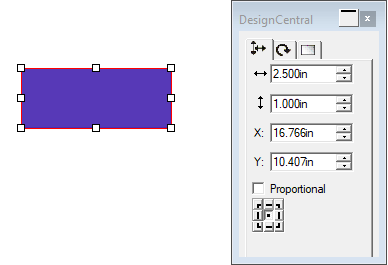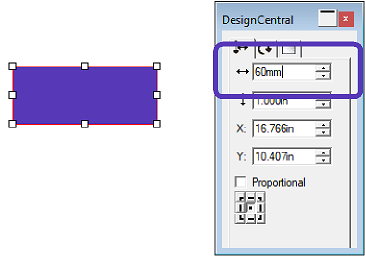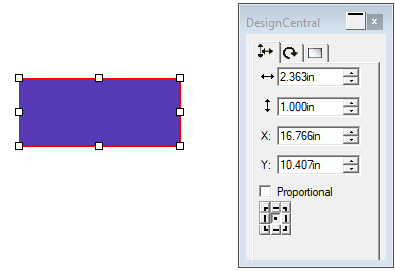# Built in Mathematical Operations

The software is able to perform a number of calculations wherever a numerical value is entered.

## Automatic Unit Conversion

If you enter a value using a different unit of measurement than the default unit, the software will automatically convert the value to the default unit.

For example, if your default unit is inches, you can enter a value of 1 ft, and the software will convert the measurement to 12 in.

Supported units are:

 in, " inch ft, ' foot mm millimeter cm centimeter m meter pt point

#### Example:

This rectangular sticker is 2.5 inches by 1 inch. My customer has asked that the sticker is exactly 60 millimeters wide.I operate in inches and don't want to change the units for the entire program. I can still change this to exactly 60mm wide.

In Design Central, I will type in "60mm." When I hit Enter, the program will do the conversion, and change it to the 60mm equivalent in inches. In this case, it will now be 2.363 inches, as shown on the right.## Simple Mathematical Operators

If you enter a simple mathematical expression, the software will calculate the result of the expression and enter that value in the field.

The available mathematical operators, in order of precedence, are:

 / Division * Multiplication + Addition - Subtraction

For example, if you enter 1/8, the value 0.125 will be calculated.

Operator precedence determines the order in which mathematical operations will be calculated when more than one operation is specified. In the table above, operators are listed from top to bottom in order of their operator precedence. For example, if you enter 6/2*3, the software will calculate 6/2 first, then multiply the result by 3, yielding a result of 9.

## Calculation of Ratios

If you enter a ratio in the format A:B, the software will scale the previous value in the field by the ratio entered.

For example, if a value is set to 12, and you enter 2:3, the new value will be 8.

## Calculation of Percentages

If you enter a percentage in the format X%, the software will scale the previous value in the field by the percentage entered.

For example, if a value is set to 10, and you enter 90%, the new value will be 9.